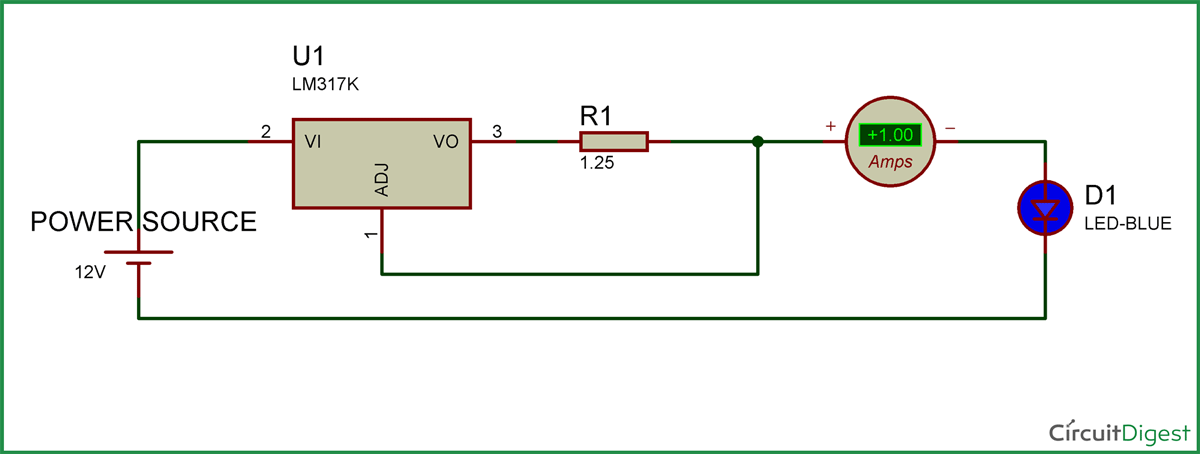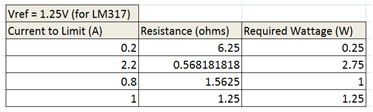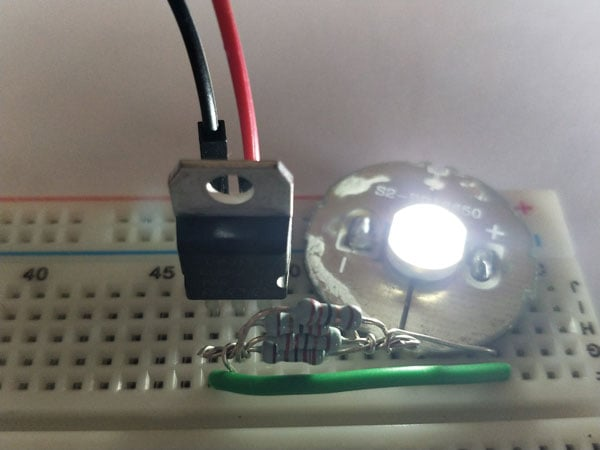High Power LED lights have lots of applications starting from Emergency lights to Head Lights in some expensive cars. These tiny little things illuminates a lot of area that we expect it to be, in this tutorial we will learn how easy it is to use one and how can we design our own circuit to drive such LEDs based in their operating current.

So let us get started.....

### Materials Required:

1. High Power LED

2. LM317

3. Resistor of desired value

### Why do we need a LED Driver circuit?

Before we get much deeper into this project, it is important to understand why one should use a LED Driver circuit to power an LED. An LED can also be powered directly form a suitable power source but in most cases the LED will pump in more current than it could handle and it eventually get destroyed. Hence we use a driver circuit which restricts the flow of current that passes through an LED. The amount of current that an LED can consume will be mentioned in the datasheet of that LED. In this article I will explain you how you can design the circuit based on your current limitations using the LM317 IC as a current limiter.

Circuit Diagram and Explanation:

The circuit Diagram of the high power LED driver is shown in the image below.As you can see the driver has nothing more than a LM317 IC and a resistor. The above circuit is designed to drive a LED with 0.2A. This current rating is fixed by the Resistor R1 in the circuit.

The formulae to determine the current is

I = Vref/R1    where, Vref is 1.25V for LM317.. so

I = 1.25/5.5

I = 0.227 Amps

As you can see in the LED driver circuit diagram, for a resistor value of 5.5 ohms the current drawn by the LED is limited to 0.23A.

You can use the same formulae and calculate the Resistor value for your desired current rating, I have calculated the values in the below table for few values of current as an example.The wattage rating of the resistor is also very important to calculate for this circuit. Because the current passing through these resistors are high. As shown in the table aove if we design a circuit for 1A then we should only use a 2 Watts resistor else the resistor will be damaged.

The wattage value of the resistor can be calculated using the below simple formulae

P = I2R

Where, I is the current that pass through the resistor and R is the resistance.

In the case of 1A, the wattage required for the resistor will be

P = 1*1*1.25 = 1.25Watts

### Working of LED Driver Circuit:

Once you have understood the circuit you can calculate the current rating required for your LED and build it on a Breadboard to test it. My circuit looks like as shown below after I built it on a breadboard.As I did not have a 5.5 ohm Resistor I used four 22 ohm resistors in parallel to get a 5.5 ohm 2Watts resistor. Most cases even you might want to do this because the resistor values will not be of standard values. In those cases, just try out some series or parallel combinations to attain your desired resistance and power rating.

The working of the project is shown in the video below. Hope you understood the project and enjoyed learning it. If you have any doubts you can reach me through the comment section or the through the forums.

Video

Subscribe below to receive most popular news, articles and DIY projects from Circuit Digest

### Related Content

•Ralph Hulslander
Aug 04, 2017

Where does the 1.25 volt come from?
In the circuit the resistor is labeled 1.25 but in the text you use a 5.5 ohm resistor.
Why not just use a 1,000 ohm resistor, wouldn't that "limit" the current?
Ralph

• Aswinth Raj
Aug 14, 2017

1.25 Volt is the reference voltage for LM317. It comes from the Ic's datasheet.

1.25 ohms is the value used in the circuit. 5.5 ohm was just used as an example.

Yes you can use a 1K ohm resistor to limit the current. Just put the value in the formulae explained above and you will know that the current will be limited to 0.00125A. Which will not be enough to run a 1W LED